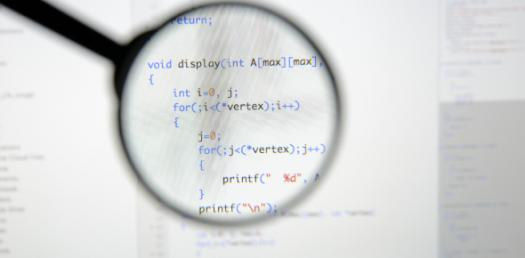# CIS 119 C Programming Quiz 1

25 Questions | Attempts: 341
ShareSettingsThis quiz is based on what we have covered so far. It also includes what was discussed on Monday class. Remember that you can take the quiz TWO times at most.

• 1.
Number 1 is a legal name for variables in C language.
• A.

True

• B.

False

• 2.
Evaluate the following code. Which variable will be assigned the value 9? int length=9, width, height;
• A.

Length

• B.

Width

• C.

Height

• D.

All of above

• 3.
The machine code is the same for computers designed by different manufacturers.
• A.

True

• B.

False

• 4.
Which of the following is NOT a strength of C language
• A.

Efficiency

• B.

Portability

• C.

Object Oriented

• D.

Flexibility

• 5.
Assembly language has a one-to-one relationship with machine code
• A.

True

• B.

False

• 6.
A typical high-level-language statement is equivalent to many machine-code instructions.
• A.

True

• B.

False

• 7.
What is the size of int in memory
• A.

4 bytes

• B.

2 bytes

• C.

8 bytes

• D.

1 bytes

• 8.
What is the only function all C programs must contain?
• A.

System()

• B.

Start()

• C.

Main()

• D.

Program()

• 9.
Which pair of punctuations is used to signal the beginning and end of a function in C?
• A.

{ and }

• B.

< and >

• C.

( and )

• D.

# and }

• 10.
Which of the following is not a correct variable type?
• A.

Float

• B.

Real

• C.

Int

• D.

Char

• 11.
In which standard library file is the function printf() located ?
• A.

Stdlib.h

• B.

Stdio.h

• C.

Stdout.h

• D.

Stdoutput.h

• 12.
In order to properly use a variable...
• A.

The variable must have a valid type.

• B.

The variable name can not be a keyword (part of the C syntax).

• C.

The variable name must begin with a letter or underscore.

• D.

All of the above.

• 13.
Which operating system was C developed for?
• A.

Windows

• B.

Solaris

• C.

Linux

• D.

Unix

• 14.
What punctuation must each statement have at the end of the line ?
• A.

:

• B.

,

• C.

!

• D.

;

• 15.
What is the output of following C program?#include int main () {     int a=6;     int b;     b=a*7/3-4*2;     printf("a=%d, b=%d\n", a, b);     return 0;}
• A.

A=6, b=20

• B.

A=6, b=12

• C.

A=6, b=16

• D.

A=6, b=6

• 16.
What is the output of the following program?main(){  int a = -5, b = -4;  printf("%d",a/b);}
• A.

1.25

• B.

-1.25

• C.

-1

• D.

1

• 17.
What is the output of the following program?void main (){int x = 10;printf ("x = %d, y = %d", x, x*2-4);}
• A.

X = 10, y = 10

• B.

X = 10, y = 9

• C.

X = 10, y = 11

• D.

X = 10, y = 16

• 18.
Which line of the following program will generate error? (The number at the left of each line indicate line number and are not part of the program code)1  #include23  int main () 4  {5      height =4;6      int height;7      printf("The height is %d\n", height);8      return 0;9  }
• A.

Line 1 and 3

• B.

Ling 5 and 6

• C.

Line 7 and 8

• D.

Line 4 and 9

• 19.
Which number is returned at the end of function to indicate that the program executes successfully?
• A.

1

• B.

0

• C.

-1

• D.

All of above

• 20.
Which symbol is used for address of the variable?
• A.

*

• B.

\$

• C.

&

• D.

@

• 21.
In a C program, the first statement that will be executed is:
• A.

The first executable statement of the program.

• B.

The first executable statement of the main() function.

• C.

The first executable statement after the comment /*start here*/

• D.

The first executable statement of the end function.

• 22.
To incldue a file that you created on your onw, single quotation marks are used. For example:   #include 'myfile.h'
• A.

True

• B.

False

• 23.
Compiler translate the middle-level and high-level language programs into equivalent assembly language programs.
• A.

True

• B.

False

• 24.
Which of the following is used to indicate that the function does not return any value?
• A.

Void

• B.

Null

• C.

No

• D.

Empty

• 25.
The type of a variable determines the operations the compiler will allow for the variable.
• A.

True

• B.

False

## Related TopicsBack to top
×

Wait!
Here's an interesting quiz for you.